JEE  >  Sample BITSAT Logical Reasoning Test

# Sample BITSAT Logical Reasoning Test

Test Description

## 10 Questions MCQ Test BITSAT Mock Tests Series & Past Year Papers | Sample BITSAT Logical Reasoning Test

Sample BITSAT Logical Reasoning Test for JEE 2023 is part of BITSAT Mock Tests Series & Past Year Papers preparation. The Sample BITSAT Logical Reasoning Test questions and answers have been prepared according to the JEE exam syllabus.The Sample BITSAT Logical Reasoning Test MCQs are made for JEE 2023 Exam. Find important definitions, questions, notes, meanings, examples, exercises, MCQs and online tests for Sample BITSAT Logical Reasoning Test below.
Solutions of Sample BITSAT Logical Reasoning Test questions in English are available as part of our BITSAT Mock Tests Series & Past Year Papers for JEE & Sample BITSAT Logical Reasoning Test solutions in Hindi for BITSAT Mock Tests Series & Past Year Papers course. Download more important topics, notes, lectures and mock test series for JEE Exam by signing up for free. Attempt Sample BITSAT Logical Reasoning Test | 10 questions in 10 minutes | Mock test for JEE preparation | Free important questions MCQ to study BITSAT Mock Tests Series & Past Year Papers for JEE Exam | Download free PDF with solutions
 1 Crore+ students have signed up on EduRev. Have you?
Sample BITSAT Logical Reasoning Test - Question 1

### Flow : River :: Stagnant : ?

Detailed Solution for Sample BITSAT Logical Reasoning Test - Question 1

As Water of a River flows similarly water of Pool is Stagnant.

Sample BITSAT Logical Reasoning Test - Question 2

### Find the missing character.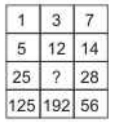Detailed Solution for Sample BITSAT Logical Reasoning Test - Question 2
• The sequence in first column is x 5. Thus, 1 x 5 = 5, 5 x 5 = 25, 25 x 5 = 125.
• The sequence in third column is x 2. Thus, 7 x 2 = 14, 14 x 2 = 28, 28 x 2 = 56.
• The sequence in second column is x 4. Thus, 3 x 4 = 12, 12 x 4 = 48, 48 x 4 = 192.

∴ Missing number = 12 x 4 = 48.

Sample BITSAT Logical Reasoning Test - Question 3

### In following number series, one term is missing find the missing term. 1,5,11,19,29,....,55

Detailed Solution for Sample BITSAT Logical Reasoning Test - Question 3

The correct sequence is + 4, + 6, + 8, + 10, ....
So, next term after 29 = 29 + 12 = 41.
The term after 41 will then be (41 + 14 = 55)
∴ 41 is missing.

Sample BITSAT Logical Reasoning Test - Question 4

Group the following figures into three classes on the basis of identical properties.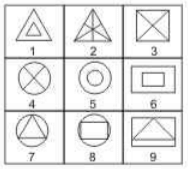Detailed Solution for Sample BITSAT Logical Reasoning Test - Question 4
• 1,5,6 have two similar elements, one inside the other.
• 2,3,4 contain straight lines each dividing the figure into two equal parts.
• 7,8,9 have one element placed inside a different element.
Sample BITSAT Logical Reasoning Test - Question 5

Complete the analogous pair.
Voter : 18 years :: Lok Sabha Member : ?

Detailed Solution for Sample BITSAT Logical Reasoning Test - Question 5

The minimum age required to vote is 18 years similarly for Lok Sabha member it is 25 years.

Sample BITSAT Logical Reasoning Test - Question 6

In this letter series, some of the letters are missing. Choose the correct letter given below -
abca _ bcaab _ ca _ bbc _ a

Detailed Solution for Sample BITSAT Logical Reasoning Test - Question 6

The correct series is abc / aabc / aabbc / aabbcc / a.
∴ Missing letters are abac

Sample BITSAT Logical Reasoning Test - Question 7

Select the correct mirror-image of the Figure (X) from amongst the given alternatives.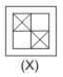Detailed Solution for Sample BITSAT Logical Reasoning Test - Question 7

Because in mirror image right becomes left and left becomes right.

Sample BITSAT Logical Reasoning Test - Question 8

Select the correct mirror-image of the Figure (X) from amongst the given alternatives.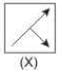Detailed Solution for Sample BITSAT Logical Reasoning Test - Question 8

Because in mirror image right becomes left and left becomes right.

Sample BITSAT Logical Reasoning Test - Question 9

Each of the following questions consists of five Problem figures marked 1,2,3,4 and 5 followed by four Answer figures marked A,B,C and D. Select a figure from the Answer figures which will continue the same series as given in the Problem figures.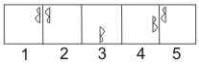Detailed Solution for Sample BITSAT Logical Reasoning Test - Question 9

The symbol gets vertically inverted and laterally inverted alternately. It also moves in anit-clockwise direction through distances equal to two half-sides (of square boundary) and three half-sides alternately.

Sample BITSAT Logical Reasoning Test - Question 10

Each of the following questions consists of five Problem figures marked 1,2,3,4 and 5 followed by four Answer figures marked A,B,C and D. Select a figure from the Answer figures which will continue the same series as given in the Problem figures.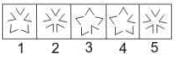Detailed Solution for Sample BITSAT Logical Reasoning Test - Question 10

Two and four 'V' shaped elements get inverted alternately.

## BITSAT Mock Tests Series & Past Year Papers

2 videos|15 docs|70 tests
 Use Code STAYHOME200 and get INR 200 additional OFF Use Coupon Code
Information about Sample BITSAT Logical Reasoning Test Page
In this test you can find the Exam questions for Sample BITSAT Logical Reasoning Test solved & explained in the simplest way possible. Besides giving Questions and answers for Sample BITSAT Logical Reasoning Test, EduRev gives you an ample number of Online tests for practice

## BITSAT Mock Tests Series & Past Year Papers

2 videos|15 docs|70 tests